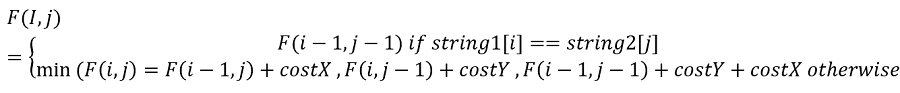# Minimum Cost to Make Two Strings Identical

Minimum Cost to Make Two Strings Identical: Here, we are going to see a standard dynamic programming (DP) problem on edit distance which can be featured in any interview rounds.
Submitted by Radib Kar, on April 22, 2020

Problem statement:

Given two strings string1 and string2 find the minimum cost required to make the given two strings identical. We can delete characters from both the strings. The cost of deleting a character from string1 is costX and string2 is costY. The cost of removing any characters from the same string is the same. Like, removing any

```    Input:
The first line contains integers costX and costY.
The second line contains the two strings X and Y.

Output:
Print the total cost to make the two strings
equal for each test case in a new line.

Constraints:
1<= length of strings X and Y <=1000
1<= costX, costY <=1000
```

Explanation of Example:

```    Input:
1
10 20
"acba" "acdb"

Output:
30

Explanation:
The similar strings would be "acb".
So need to remove one from string1 and one from string2
costing total of 30.
```

Solution Approach:

The problem can be solved recursively,

Let,

```    N = length of string1
M = length of string1
F(n,m) = minimum cost to make two strings similar
```

Let us consider, what can be cases that can arrive for F(i,j) where 0 <= i<n && 0 <= j<m

Case 1.

string1[i]==string2[j] that is indexed characters are similar

In such a case, we don't need any additional cost to make strings similar, the cost will be similar to sub-problem of size i-1, j-1. This can be recursively written as

```    F(i,j) = F(i-1,j-1) if string1[i] == string2[j]
```

Case 2.

string1[i]!=string2[j] that is indexed characters are not similar.

In such a case we need to invest,

1. One thing can remove the indexed character from string1 and change to indexed string2 character.
This can be recursively written as,
```    F(i,j) = F(i-1,j) + costX if string1[i] != string2[j]
```
2. Another way can be to remove the indexed character from string2 and change to string1 indexed character.
This can be recursively written as,
```    F(i,j) = F(i,j-1) + costY if string1[i] != string2[j]
```
3. Remove characters from both.
This can be recursively written as,
```    F(i,j) = F(i-1,j-1) + costY + costX if string1[i] != string2[j]
```
Finally, we would take minimum out of this three cases.

So here goes the problem structure,Now the above recursion will create many overlapping subproblems and hence we need two converts it into DP.

Converting into DP

```    n = string1 length
m = string2 length
str1 = string1
str2 = string2

1)  Declare int dp[n+1][m+1];
2)  Base case
for i=0 to n
dp[i]=i*costX;
for j=0 to m
dp[j]=j*costY;
3)  Fill the DP
for i=1 to n
for j=1 to m
//first case when str1[i-1]==str2[j-1]
dp[i][j]=dp[i-1][j-1];
if(str1[i-1]!=str2[j-1])
dp[i][j]+=costX+costY;
dp[i][j]=min(dp[i][j],min(dp[i-1][j]+costX,dp[i][j-1]+costY));
end if

end for
end for
4)  return dp[n][m];
```

C++ Implementation:

```#include <bits/stdc++.h>
using namespace std;

int editdistance(string s1, string s2, int costX, int costY)
{

int n = s1.length();
int m = s2.length();
int dp[n + 1][m + 1];

for (int i = 0; i <= n; i++)
dp[i] = i * costX;

for (int j = 0; j <= m; j++)
dp[j] = j * costY;

for (int i = 1; i <= n; i++) {
for (int j = 1; j <= m; j++) {
dp[i][j] = dp[i - 1][j - 1];
if (s1[i - 1] != s2[j - 1]) {
dp[i][j] += costX + costY;
dp[i][j] = min(dp[i][j], min(dp[i - 1][j] + costX, dp[i][j - 1] + costY));
}
}
}

return dp[n][m];
}

int main()
{
int costX, costY;

cout << "Cost of changing/removing character from string1: \n";
cin >> costX;

cout << "Cost of changing/removing character from string2: \n";
cin >> costY;

string s1, s2;

cout << "Input string1\n";
cin >> s1;
cout << "Input string2\n";
cin >> s2;

cout << "Minimum cost to make two string similar: " << editdistance(s1, s2, costX, costY) << endl;

return 0;
}
```

Output

```Cost of changing/removing character from string1:
2
Cost of changing/removing character from string2:
3
Input string1
includehelp
Input string2
includuggu
Minimum cost to make two string similar: 22
```